# Java Program to print Floyd’s triangle

In this tutorial you will learn about the Java Program to print Floyd’s triangle and its application with practical example.

## Java Program to print Floyd’s triangle.

In this tutorial, we will learn to create a Java Program to print Floyd’s triangle using Java programming.

## Prerequisites:-

Before starting with this tutorial we assume that you are best aware of the following Java programming topics:

• Operators in Java Programming.
• Basic Input and Output function in Java Programming.
• Basic Java programming.
• For loop in Java Programming.

## Floyd’s Triangle:-

Floyd’s triangle is a right-angled triangle that contains mathematical numbers from 1 and goes up to the required point.

## Printing a Floyd’s:-

The Java language is a very powerful programming language. In Java programming, we can perform many operations with the help of coding’s. Java is a very easy language to create any shape. In this program, we will learn to create Floyd’s triangle with the help of some code.

## Program:-

To print a Floyd’s Triangle

## Output:-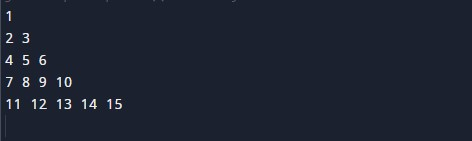In the above program, we have first initialized the required variable.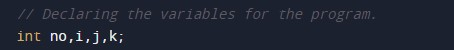• i = it will hold the integer value to control parent for loop.
• j = it will hold the integer value to control child for loop.
• k = it will hold the integer value to control child for loop.
• no = it will hold the integer value for the number of rows.

Taking input Number of rows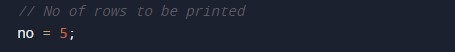Nested For loop the body of the program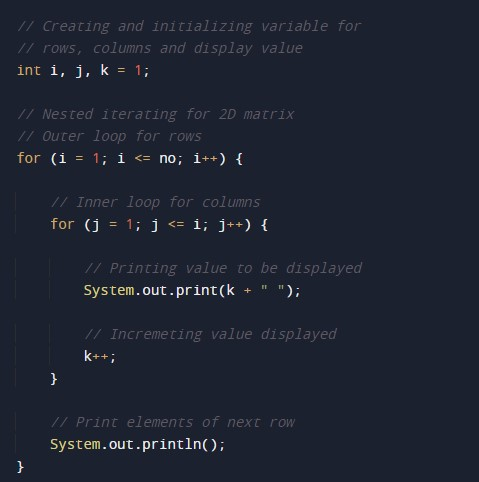Printing Numbers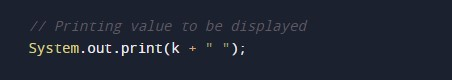In this tutorial we have learn about the Java Program to print Floyd’s triangle and its application with practical example. I hope you will like this tutorial.